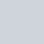Livio/ June 29, 2020/ Power BI, Power Query/ 1 comments

Recursive Functions in Power Query

We are all more or less familiar about recursive functions with programming languages such as Python, but what about Power Query? Can we use recursive functions with it? The answer is YES. This post will walk you through an easy example.

An easy example: Factorial Function

The first example involves writing a simple factorial function. The function is shown below:

As you can see, it contains two blocks of let/in. The outer block declares and returns the function, while the inner blocks defines its functionality. The recursive call to the function is done by prefixing the name of the function with a @Power Query Recursive Function

A more useful example: Traversing a Hierarchy

While the previous example was a good learning exercise, it was not so useful since Power Query already has a native Number.Factorial function written for you.

Let us look at a better example! Imagine you had the below table and wanted to add a custom column representing the ID of the individual at the very top of the hierarchy. It becomes quite easy using a recursive function:Power Query Recursive Function

Adding a custom column using the above function will give us the below results:Power Query Recursive

Of course you are not limited to this, you can also return a list of IDs in the hierarchy:Power Query Recursive Function

Conclusions

Learning to use recursive functions in Power Query opens up the door to solving new problems, trying things you may have not thought about before.

Did you find this post useful? Would you like to see more examples? Have you used recursive functions in Power Query before? Please share it and leave a comment down below! See you at the next post!

## 1 Comment

1.Hi,
Thanks for the article.
I came across a problem if IDs in hierarchy are not in ascending order (e.g. “1 > 99 > 4” would return corresponding names as if it was “1 > 4 > 99” hierarchy). Is there a tweak to overcome this?